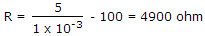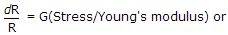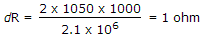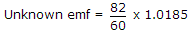# Electronics and Communication Engineering - Measurements and Instrumentation

### Exercise :: Measurements and Instrumentation - Section 1

26.

Magnetic flux density has the dimensions

 A. MI-1T-2 B. M-1I-2T-2 C. ML-3T-2 D. MI-1T-3

Explanation:

Flux density = flux/area.

27.

A meter with a resistance of 100 Ω and a full scale deflection of current of 1 mA is to be converted into voltmeter of 0 - 5 V range. The multiplier resistance should be

 A. 490 Ω B. 5000 Ω C. 4900 Ω D. 5100 Ω

Explanation:.

28.

In a resistance strain gauge, G = 2, stress = 1050 kg/cm2, R = 1000 Ω. The value of ΔR will be

 A. 2 Ω B. 3 Ω C. 4 Ω D. 1 Ω

Explanation:.

29.

The household energy meter is

 A. indicating instrument B. recording instrument C. integrating instrument D. none of the above

Explanation:

It integrates energy over a period of time.

30.

A standard cell of 1.0185 V is used with a slide wire potentiometer. The balance was obtained at 60 cm. When an unknown emf was connected, the balance was obtained at 82 cm. The magnitude of unknown emf is

 A. 1.39 V B. 0.75 V C. 13.9 V D. 7.45 V.